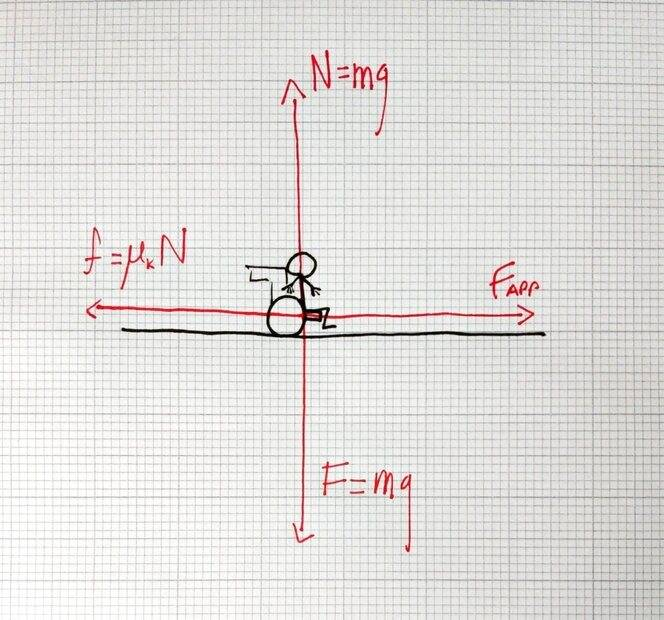# Total work done while pushing a wheelchair

TheBigDig
Homework Statement:
A person in a wheelchair (a total mass of 100 kg) is pushed from rest to a
speed of 2.0 m/s . If the effective coefficient of friction is 0.05 and the
distance moved is 15 m, what is the total work done?
Relevant Equations:
##v^2 = u^2 +2as##
## F = ma ##
##f = \mu_k N##
##W = Fs##Taking v = 2m/s, u=0m/s and s = 15m, we get
##a=0.13m/s^2##
##F_g = mg = 100(9.8) = 980N##
Since there's no vertical acceleration, the normal force is equal to the weight
##N = 980N##

##f = \mu_k N = 0.05(980) = 49N##
##F_{net} = ma = 100(0.13) = 13N##
##F_{app} = F_{net}+f = 62N##

My question is, is my work done the applied force by the distance or the net force by the distance. I'm a little unsure of it.

Homework Helper
Gold Member
2022 Award
My question is, is my work done the applied force by the distance or the net force by the distance. I'm a little unsure of it.
Good question! It's not clear which force to assume.

The other thing is that wheels when rolling work by static friction, so technically there is no relevant coefficient of kinetic friction and no energy loss to kinetic friction. Instead, there will be some rolling resistance. To see the difference, compare pushing the wheelchair normally (low rolling resistance) and pushing the wheelchair when it has the wheels locked by the brakes (high kinetic friction - to the point where the wheelchair is perhaps immovable with someone in it and the wheels locked).

This is perhaps overall a bad question.

Homework Helper
Gold Member
2022 Award
Homework Statement:: A person in a wheelchair (a total mass of 100 kg) is pushed from rest to a
speed of 2.0 m/s . If the effective coefficient of friction is 0.05 and the
distance moved is 15 m, what is the total work done?
Relevant Equations:: ##v^2 = u^2 +2as##
## F = ma ##
##f = \mu_k N##
##W = Fs##

View attachment 292266
Taking v = 2m/s, u=0m/s and s = 15m, we get
##a=0.13m/s^2##
##F_g = mg = 100(9.8) = 980N##
Since there's no vertical acceleration, the normal force is equal to the weight
##N = 980N##

##f = \mu_k N = 0.05(980) = 49N##
##F_{net} = ma = 100(0.13) = 13N##
##F_{app} = F_{net}+f = 62N##

My question is, is my work done the applied force by the distance or the net force by the distance. I'm a little unsure of it.
One critcism of your solution is that you had to assume constant acceleration in order to use the SUVAT formulas. Instead, the gain in KE of the wheelchair tells you the work done to accelerate it without considering the applied force or acceleration profile at all. Likewise, the effective friction force times the distance would have given you the negative work done by friction.